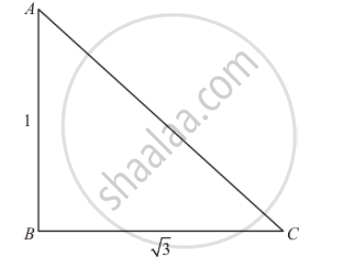Advertisement Remove all ads

# If the Angle of Elevation of a Tower from a Distance of 100 Metres from Its Foot is 60°, Then the Height of the Tower is - Mathematics

MCQ

If the angle of elevation of a tower from a distance of 100 metres from its foot is 60°, then the height of the tower is

#### Options

•  100$\sqrt{3}$

• $\frac{100}{\sqrt{3}} m$

• $50 \sqrt{3}$

• $\frac{200}{\sqrt{3}} m$

Advertisement Remove all ads

#### Solution

Let AB be the height of tower is h metersGiven that: angle of elevation is 60° from tower of foot and distance BC=100 meters.

Here, we have to find the height of tower.

So we use trigonometric ratios.

In a triangle ABC

⇒ tan C= (AB)/(BC)

⇒tan 60°= (AB)/(BC)

⇒ sqrt3= h/100

⇒ h= 100sqrt3

Is there an error in this question or solution?
Advertisement Remove all ads

#### APPEARS IN

RD Sharma Class 10 Maths
Chapter 12 Trigonometry
Q 2 | Page 41
Advertisement Remove all ads

#### Video TutorialsVIEW ALL 

Advertisement Remove all ads
Share
Notifications

View all notifications

Forgot password?
Course